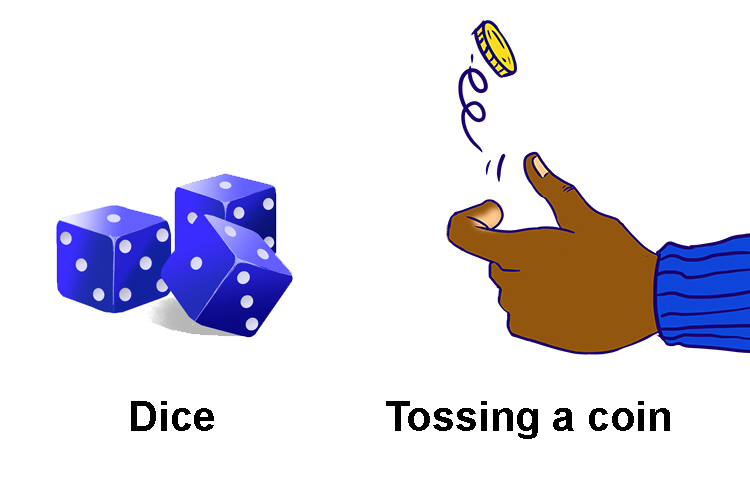# Highest number and lowest number it can be

The highest and lowest number probability
USE LOGIC

Remember our two most famous probability examples:probability=1/6=0.167                                                           probability=1/2=0.5

But what would these examples be if you could have the perfect outcome, the perfect probability.

## What’s the largest number this could be if you have the perfect outcome each time?

Probability = (The\ n\umber\ of\ RIGHT\ answers)/(The\ n\umber\ of\ALL\  possibl\e\ answers

Probability = 1/1       \     \       \    ((on\e\ right\ answer))/((only\ on\e\ possibl\e\ solution\))=1

This is the most successful probability can be i.e. 1

i.e. Certain probability get correct answer = 1

## What’s the smallest number this could be if you have the worst outcome each time?

Probability = (The\ n\umber\ of\ RIGHT\ answers)/(The\ n\umber\ of\ALL\  possibl\e\ answers

Probability =(on\e\ right\ answer)/(Lets\ say\ 1\ million\ possibilities\)=0.000,001

approx\Zero

i.e. absolute probability of losing would be answer = zero

Summary:

Certain to happen = 1

Certain NOT to happen = 0

NOTE:

The probability of an event not occurring is 1 (certain probability) minus the probability of it occurring.

Example:

If there was a 0.4 probability of rain (40% chance of rain), there is a 0.6 probability it will not rain (60% chance rain won’t occur). (1 - 0.4 = 0.6).